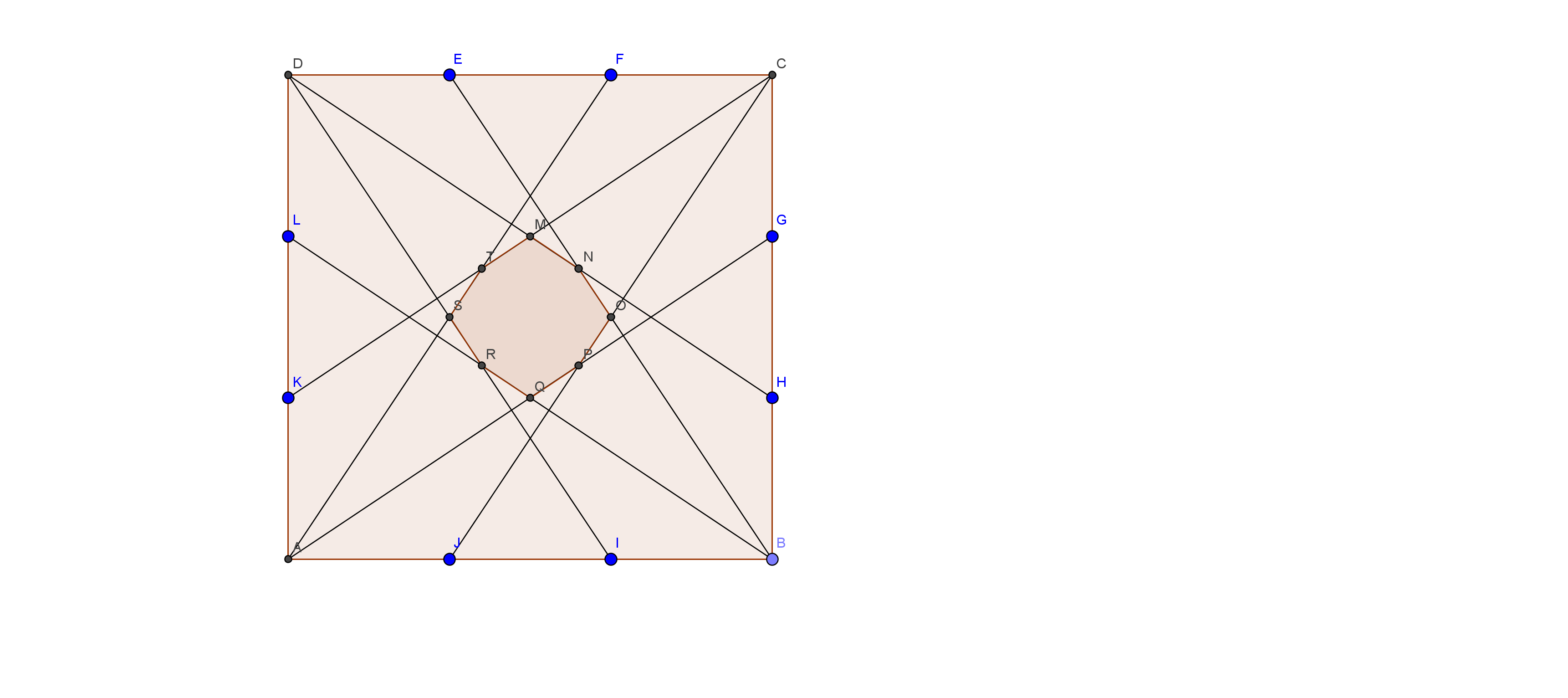# Midpoint? What About Thirds? - Part 3

Geometry Level 4As usual, $ABCD$ is a square, and we divide each side​ into three equal parts, and mark the points $E,F,G,H,I,J,K,L$ in a clockwise manner starting from the vertex $D$. This time, we connect those points to the furthest vertex that is opposite to them to form a not-necessarily-regular octagon as shown in the figure above, for example, points $B$ and $E$ will be connected in this case. What is the ratio of the area of the square to that of the octagon?

×